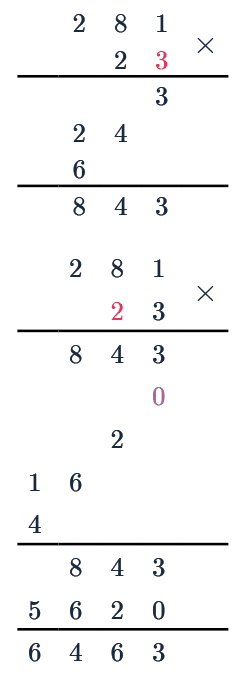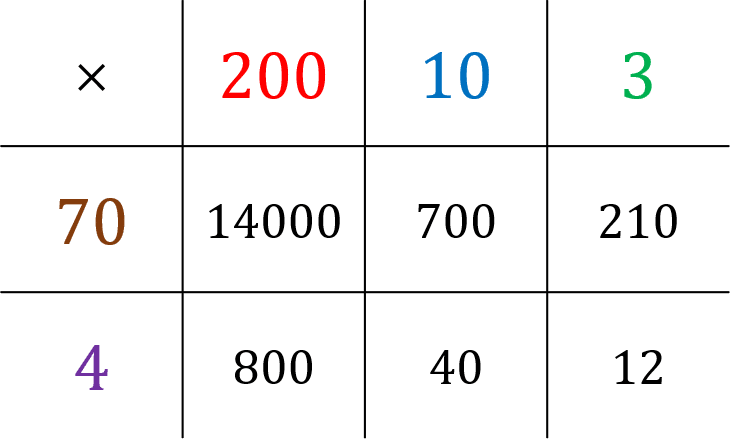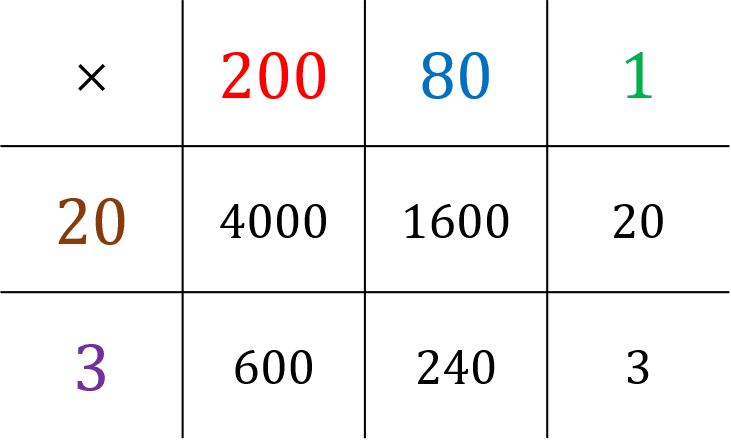# Long Multiplication

GCSEKS3Level 1-3AQAEdexcelOCRWJEC

## Long Multiplication and Grid Method

The 2 multiplication methods we will see are the long multiplication (column) method and the grid method. The grid method is taught by many schools in the UK but there are many that prefer long multiplication, so we will look at both.

Level 1-3GCSEKS3AQAEdexcelOCRWJEC## Method 1: Long Multiplication (Column) Method

Step 1: Write the two numbers we are multiplying on top of each other, usually with the bigger one on top. We write the values so that the $1$s, $10$s, $100$s and so on all line up in the correct column.

Step 2: Multiply the last digit from the bottom number with every digit from the top number, writing the results underneath from right to left, and carrying forward when values go above $10$.

$3\times1 = 3$, $3\times8=24$, $3\times2 = 6$

Step 3: Next, the second digit is the tens digit, for this we write a $0$ underneath the last step’s working so that everything is shifted one space to the left.

We then multiply this digit by every digit from the top number, writing the results underneath the last step from right to left.

Step 4: Add up the final numbers using the column addition method, writing the results under another line.

From this we can see:

$281 \times 23 = 6463$

Level 1-3GCSEKS3AQAEdexcelOCRWJEC
Level 1-3GCSEKS3AQAEdexcelOCRWJEC

## Method 2: The Grid MethodStep 1: Split each number into $1$s, $10$s, $100$s and so on, and write each component of one number along the top of a grid, and each component of the other number down the left-hand side.

$\textcolor{red}{2}\textcolor{blue}{1}\textcolor{limegreen}{3} \times \textcolor{maroon}{7}\textcolor{purple}{4}$

Step 2: Fill all the squares of the grid by multiplying each part of one number by each part of the other.

$14000+800+700+210+40+12=15762$

Level 1-3GCSEKS3AQAEdexcelOCRWJEC

## Example: The Grid MethodWork out $23\times 281$, without using a calculator.

[3 marks]

Split each number into $1$s, $10$s, $100$s and so on, writing each component of one number along the top of the grid, and each component of the other number down the left-hand side. Then, fill all the squares of the grid by multiplying each bit of one number by each bit of the other number.

Then, by whichever method you prefer, add together all the answers in the grid.

$4000+1600+600+240+20+3=6463$

So,

$281 \times 23 = 6463$

Level 1-3GCSEKS3AQAEdexcelOCRWJEC

## Long Multiplication Example Questions

For this multiplication we will use the long multiplication method, multiplying $619$ first by $5$ then by $40$,

$\begin{array}{r}619\\\times45\\\hline3095\\24760\end{array}$

Adding the results of each components multiplication,

$\begin{array}{r}\begin{array}{r}3095\\+24760\\\hline27855\end{array}\end{array}$Gold Standard Education

For this multiplication we will use the long multiplication method, multiplying $52$ first by $1$ then by $30$,

$\begin{array}{r}\begin{array}{r}52\\\times31\\\hline52\\1560\end{array}\end{array}$

Adding the results of each components multiplication,

$\begin{array}{r}\begin{array}{r}52\\+1560\\\hline1612\end{array}\end{array}$Gold Standard Education

For this multiplication we will use the long multiplication method, multiplying $760$ first by $4$ then by $20$,

$\begin{array}{r}\begin{array}{r}760\\\times24\\\hline3040\\15200\end{array}\end{array}$

Adding the results of each components multiplication,

$\begin{array}{r}\begin{array}{r}3040\\+15200\\\hline18240\end{array}\end{array}$Gold Standard Education

For this multiplication we will use the long multiplication method, multiplying $364$ first by $2$ then by $50$,

$\begin{array}{r}\begin{array}{r}364\\\times52\\\hline728\\18200\end{array}\end{array}$

Adding the results of each components multiplication,

$\begin{array}{r}\begin{array}{r}728\\+18200\\\hline18928\end{array}\end{array}$Gold Standard Education

For this multiplication we will use the long multiplication method, multiplying $185$ first by $8$ then by $30$,

$\begin{array}{r}\begin{array}{r}185\\\times38\\\hline1480\\5550\end{array}\end{array}$

Adding the results of each components multiplication,

$\begin{array}{r}\begin{array}{r}1480\\+5550\\\hline7030\end{array}\end{array}$